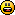# Math Is Fun Forum

Discussion about math, puzzles, games and fun.   Useful symbols: ÷ × ½ √ ∞ ≠ ≤ ≥ ≈ ⇒ ± ∈ Δ θ ∴ ∑ ∫  π  -¹ ² ³ °

You are not logged in.

## #1 2023-02-24 09:40:33

paulb203
Member
Registered: 2023-02-24
Posts: 20

### Why are inequalities so called?

Why does, for example, 2y+1 is less than or equal to 7 count as an inequality?
If x is 3 then 2y+1 is equal to 7, not ‘inequal’, yeah?

Offline

## #2 2023-02-24 13:35:58

Jai GaneshRegistered: 2005-06-28
Posts: 42,257

### Re: Why are inequalities so called?

Hi paulb203,

Solving Inequalities

It appears to me that if one wants to make progress in mathematics, one should study the masters and not the pupils. - Niels Henrik Abel.

Nothing is better than reading and gaining more and more knowledge - Stephen William Hawking.

Offline

## #3 2023-02-25 07:36:19

paulb203
Member
Registered: 2023-02-24
Posts: 20

### Re: Why are inequalities so called?

Thanks, ganesh.
I've worked my way through those pages already; very helpful. But the question I posted remained afterwards.
It seems to me to be a misnomer ('inequality') given that the two quantities involved are not always necessarily inequal, e.g, the example I gave in my original post, 2y+1 can be equal to 7 when y is 3
(I made a mistake in the original post - I put x when I meant y).

Offline

## #4 2023-02-25 21:44:27

BobRegistered: 2010-06-20
Posts: 9,649

### Re: Why are inequalities so called?

hi paulb203

Welcome to the forum.

In an equation two expressions (LHS and RHS) are said to be equal in value.  There's a whole set of techniques for solving problems using equations.  An equation may have more than one solution.

Sometimes a whole range of values may form the solution to a problem.  In such cases an algebraic 'thing' is created that is not an equation.  This is where the word inequality is used.  It's just the name for such a thing so don't get hung up on why it is called that. Let's see an example.

A company charges a fix amount, say \$10, to deliver goods plus the cost of the goods. Let's say they cost \$5 each.

So an expression for the charge would be 10 + 5n where n is the number of items being bought.

Now I'll create a problem that uses an inequality.  Let's say that when the total cost exceeds \$100 the company wants payment in advance whereas at \$100 and below they'll accept cash on delivery.  What's the n value when payment in advance kicks in?

The expression for the total cost was 10 + 5n and the in advance payment happens when this is over \$100.  So I can write

10 + 5n > 100

You can solve this just like an equation.

Subtract 10 from each side:

5n > 90

divide by 5:

n > 18

So a customer will have to pay in advance if they want to buy more than 18 items (ie. 19, 20 etc)

If I change the sentance to  when the total cost is \$100 or above the company wants payment in advance, then the inequality becomes

10 + 5n  ≥ 100 and the solution is now n ≥ 18 meaning that the rule kicks in at 18, rather than above 18.

There just one special rule for dealing with inequalities that relates to what we mean by >

On the number line ( negatives on the left, positives on the right) A > B means A is further to the right on the line than B.  So

6 > 3
-3 > -6

When using algebraic rules to solve an inequality you might have a situation where you multiply or divide by a negative amount.  This has the effect of reflecting the LHS and RHS through zero.  This means the inequality sign must change.

example.  6 > 3  multiply by -1 and it becomes -6 < -3.  If you don't switch the sign like this your algebra will go wrong.

Example.

10 - 3x > 4

If I solve by avoiding multiplying/or dividing by a negative I get this correct solution
10 > 4 + 3x

subtract 4
10 - 4 > 3x

6 > 3x

divide by 3
2 > x.  We can re-write this as x < 2 ;  it means the same thing.

Look what happens if I solve this way:

10 - 3x > 4
subtract 10
-3x > -6
Divide by -3 but forgetting to switch the sign

x > 2

This we alreacy know is not correct.  So you can appreciate why the sign must be switched too.

X < 2 is correct.

Hope this helps,

Bob

Children are not defined by school ...........The Fonz
You cannot teach a man anything;  you can only help him find it within himself..........Galileo Galilei
Sometimes I deliberately make mistakes, just to test you!  …………….BobOffline

## #5 2023-02-26 08:25:40

paulb203
Member
Registered: 2023-02-24
Posts: 20

### Re: Why are inequalities so called?

Thanks a lot, Bob. That was very helpful. I had fun working out the 10+5n example before looking at the answer.
I did;
100-10=90
90/5=18
But I couldn't come up with the expression 10+5n is greater than 100)

I had noted the warning about changing the sign from the examples on the main website but your examples were good revision. Thanks.

Offline

## #6 2023-02-26 08:27:04

paulb203
Member
Registered: 2023-02-24
Posts: 20

### Re: Why are inequalities so called?

Ha! My emoji was supposed to be a smiley faceOffline